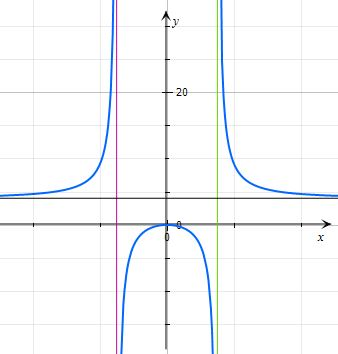# Sketch the graph of f(x) = 4x^2 / x^2-9 showing any asymptotes, intercepts, increasing /...

## Question:

Sketch the graph of {eq}\displaystyle f(x) = \frac {4x^2} {x^2-9} {/eq} showing any asymptotes, intercepts, increasing / decreasing, extrema, concavity, and points of inflection.

## Graphing functions:

The different types of functions vary greatly in their graph form. Usually, we would be able to tell the type of function by looking at the graph. In our problem, we have a rational function, a kind of function that is a ratio between two polynomials.

## Answer and Explanation:

Below, we can see the graph of the function {eq}\displaystyle f(x) = \frac{4x^2}{x^2-9} {/eq}. We will be able to see the asymptotes, intercepts, increasing / decreasing, extrema, concavity, and points of inflection in the graph:The asymptotes are at {eq}\displaystyle x= \pm 3, y = 4 {/eq}, and the intercept is at {eq}\displaystyle (0,0) {/eq}.

#### Learn more about this topic:Graphs: Types, Examples & Functions

from High School Algebra II: Help and Review

Chapter 16 / Lesson 11
355K